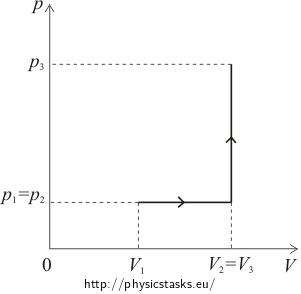## Work, Heat and the Internal Energy Difference of Oxygen

### Task number: 1796

An initial volume of oxygen is 5 l and its pressure is 100 kPa. First the oxygen was isobarically heated to the double volume, then we have isochorically increased the pressure four times its initial pressure. Determine the work performed by the gas, the heat we had to supply to the gas and its internal energy difference.

• #### Notation

 V1 = 5 l = 0.005 m3 initial volume of oxygen p1 = 100 kPa = 105 Pa initial pressure of oxygen V2 = 2V1 volume after heating p3 = 4p1 isochorically increased pressure W = ? performed work Q = ? supplied heat ΔU = ? internal energy difference
• #### pV diagram• #### Hint no.1 – calculating the heat

To calculate the heat supplied during the isobaric process, use the relation between the heat Q and molar heat capacity at constant pressure Cp

$Q=nC_p\mathrm{\Delta}T,$

where n is the amount of substance and ΔT is the gas temperature difference.

To calculate the heat supplied during the isochoric process, use the analogous relation between the heat Q and molar heat capacity at constant volume CV

$Q=nC_V\mathrm{\Delta}T,$

where n is the amount of substance and ΔT is the gas temperature difference.

• #### Hint no. 2 – molar heat capacity of oxygen

Molar heat capacity of oxygen at constant pressure is

$C_p=\frac{7}{2}R.$

Molar heat capacity of oxygen at constant volume is

$C_V=\frac{5}{2}R.$
• #### Hint. no 3 – expressing the temperature change

To express the temperature change during the isobaric process, use so-called Gay-Lussac’s law and for the isochoric process use so-called Charles’ law.

• #### Analysis – supplied heat

Total heat supplied to the gas is equal to the sum of the heat supplied during the isobaric process and the heat supplied during the isochoric process.

We express the heat supplied during the isobaric process as the product of the amount of substance, molar heat capacity at constant volume and temperature change during the process. We determine the temperature change using so-called Gay-Lussac’s law. Finally, we use the classical ideal gas law, so we can express the heat using given physical quantities.

To calculate the heat supplied during the isochoric process, we proceed in an analogous way. However, to express this heat, we use the molar heat capacity at constant volume and determine the temperature change using so-called Charles’ law.

• #### Calculating supplied heat

We supply heat to the gas during both processes, therefore for the total heat Q supplied to the gas it holds that

$Q=Q_1+Q_2,$

where Q1 and Q2 stands for the heat supplied during the isobaric and isochoric process respectively.

To calculate the heat Q1 supplied during the isobaric process we use the relation between the heat and molar heat capacity at constant pressure Cp

$Q_1=nC_p\left(T_2-T_1\right),$

where n is the amount of substance, T2T1 are the final and initial temperature of the gas.

Considering the fact, that oxygen is a diatomic gas, thus its molar heat capacity at constant pressure is

$C_p=\frac{7}{2}R,$

we can write

$Q_1=\frac{7}{2}nR\left(T_2-T_1\right).$

According to Gay-Lussac’s law it holds that if the volume isobarically increases twofold, the temperature increases twofold as well, therefore T2 = 2T1. Substituting it into the previous relation, we obtain

$Q_1=\frac{7}{2}nR\left(2T_1-T_1\right)=\frac{7}{2}nRT_1.$

Now we are going to use the ideal gas law

$p_1V_1=nRT_1 \qquad\Rightarrow\qquad nT_1=\frac{p_1V_1}{R}.$

By substitution we obtain the relation

$Q_1=\frac{7}{2}R\frac{p_1V_1}{R}=\frac{7}{2}p_1V_1.$

For the heat Q2 supplied during the isochoric process it holds that

$Q_2=nC_V\left(T_3-T_2\right).$

Molar heat capacity of oxygen at constant volume is

$C_V=\frac{5}{2}R,$

thus we can write

$Q_2=\frac{5}{2}nR\left(T_3-T_2\right).$

According to Charles’ law for isochoric process it holds that if the pressure increases fourfold, the temperature increases fourfold as well, therefore T3 = 4T2 = 8T1.

Substituting it into the previous relation

$Q_2=\frac{5}{2}nR\left(8T_1-2T_1\right)=15nRT_1$

and reusing the ideal gas law

$nT_1=\frac{p_1V_1}{R},$

we obtain the relation for the heat Q2

$Q_2=15R\frac{p_1V_1}{R}=15p_1V_1.$

Then the total heat Q supplied to the gas is

$Q=Q_1+Q_2=\frac{7}{2}p_1V_1+15p_1V_1,$ $Q=\frac{37}{2}p_1V_1.$
• #### Hint no. 4 – calculating the work

Work performed by the gas is non-zero only during the isobaric heating and for this work it is true that

$W=p\left(V_2-V_1\right),$

where p is the pressure of the gas, V1 and V2 are volumes of the gas before heating and after that.

During the isochoric process the volume of the gas is constant, therefore the gas does not perform work.

• #### Hint no. 5 – the internal energy difference

To calculate the internal energy difference ΔU, use the first law of thermodynamics.

• #### Analysis – performed work and the internal energy difference

First of all, we have to realize that the gas performs work only during the isobaric heating. This work we express as the product of the pressure and volume change of the gas during the process.

We determine the internal energy difference from the first law of thermodynamics.

• #### Calculating performed work and the difference in internal energy

Work W performed by the gas is non-zero only during the isobaric heating and for this work it is true that

$W=p_1\left(V_2-V_1\right),$

where p1 is the pressure of the gas, V1 and V2 are volumes of the gas before heating and after that.

After substituting the volume V2

$W=p_1\left(2V_1-V_1\right)$

we promptly obtain

$W=p_1V_1.$

We determine the internal energy change ΔU from the first law of thermodynamics as the difference between the supplied heat Q and performed work W

$\Delta U=Q-W=\frac{37}{2}p_1V_1 -p_1V_1= \frac{35}{2}p_1V_1.$
• #### Numeric substitution

$Q=\frac{37}{2}p_1V_1=\frac{37}{2}\cdot{0.005}\cdot{10^5}\,\mathrm{J}=9250\,\mathrm{J}=9.25\,\mathrm{kJ}$ $W=p_1V_1=0.005\cdot{10^5}\,\mathrm{J}=500\,\mathrm{J}$ $\Delta U=Q-W=\left(9250-500\right)\,\mathrm{J}=8750\,\mathrm{J}=8.75\,\mathrm{kJ}$
• #### Answer

The gas performed work of 500 J, the heat supplied to the gas was 9.25 kJ and increase of its internal energy was 8.75 kJ.